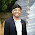## Thursday, July 26, 2012

### Rudin-Osher-Fatemi image denoising model

Today we will finally touch the subject of the celebrated image denoising model proposed by Rudin, Osher and Fatemi in 1992, ( L. Rudin, S. Osher, E. Fatemi, "Nonlinear total variation based noise removal algorithms", Physica D, vol. 60, pp. 259–268, 1992.)

This model is motivated by the following problem in image restoration:
Given a noisy image $f:\Omega\rightarrow \mathbb{R}$, where $\Omega$ is bounded open subset of $\mathbb{R}^2$, we need to obtain a clean image $u$.

For solving this problem they proposed the following minimization problem:
$$\mbox{ minimize } \int_{\Omega}|\nabla u|\\\mbox{ subject to } \int_{\Omega} u=\int_{\Omega}f \, \hspace{10 pt}\mbox{ and } \hspace{10 pt}\int_{\Omega} |f-u|^2 = \sigma^2.$$ where $\sigma^2$ is the estimated global variance of the additive noise. This can be also formulated in terms of soft constraint minimization:
$$u_{\lambda}=\arg\min_u \{\,|u|_{BV(\Omega)} + \lambda \Vert f-u\Vert_{L^2(\Omega)}^2\}.$$ where $|u|_{BV(\Omega)}$ is the BV-seminorm.
In general, this belongs to the class of minimization problems of the following type
$$u_{\lambda}=\arg\min_u \{\,J(u) + H(f, u) \}$$ where $J$ is a convex nonnegative regularization functional and the fitting functional $H$ is convex nonnegative with respect to $u$ for fixed $f$. Due to convexity there exists a minimizer $u_{\lambda}$ such that '0' is in the sub-differential of $J(u) + H(f, u)$ at $u_{\lambda}$ i.e.
$$0\in\partial_u J(u_{\lambda})+\partial_u H(u_{\lambda}, f).$$
(here subdifferential $\partial_u J$ at $u_{\lambda}$ is the set $\partial_u J(u_{\lambda}):=\{v:J(u)\geq J(u_{\lambda})+\langle v, u-u_{\lambda} \rangle \}$. )

If $H(u, f)=\lambda\Vert f-u\Vert_{L^2(\Omega)}^2$, then $\partial_u H(u, f)=2\lambda (u-f)$. Moreover if $H(u, f)=\lambda\Vert f-Ku\Vert_{L^2(\Omega)}^2$, where $K:L^2(\Omega)\rightarrow \mathcal{H}$, $\mathcal{H}$ being some Hilbert space, then $\partial_u H(u, f)=2\lambda K^*(Ku-f)$, where $K^*$ denotes an adjoint of $K$.

Formally, we can write $\partial J(u)=-\mbox{div}\Big( \frac{\nabla u}{|\nabla u|}\Big)$, and we can write the Euler-Lagrange differential equation for the Rudin Osher Fatemi model as follows:
$$0=-\mbox{div}\Big( \frac{\nabla u}{|\nabla u|}\Big)+2\lambda(u-f)$$ or equivalently,
$$u=f+\frac{1}{2\lambda}\mbox{div}\Big( \frac{\nabla u}{|\nabla u|}\Big)$$
We impose Neumann boundary condition $\nabla u\cdot\nu=0$ in the boundary $\partial \Omega$, this ensures the condition on the mean: $\int_{\Omega}u=\int_{\Omega}f$.

Dependence on the scaling parameter $\lambda$:

The parameter $\lambda$ determines the denoising level. If $\lambda$ is small, then it is equivalent to penalizing the fidelity term more and the minimizer $u_{\lambda}$ is very close to the original image. On the other hand, for large $\lambda$ the minimizer is a cartoon representation of the image $f$.

We perform a few experiments on the image of Lenna:Original image, Lena.

For $\lambda=0.8$ and the image in the range [0, 255] we get the following result:The denoised image $u_{\lambda}$ for $\lambda=0.8$
We see above that for this $\lambda=0.8$ the denoising is minimal.

For $\lambda=0.05$ and the image in the range [0, 255] we get the following result:The denoised image $u_{\lambda}$ for $\lambda=0.05$.

Here we see the details are gone, but we still see a lot of structure. Let's see what happens if we take $\lambda=0.01$, with image in [0, 255].The denoised image $u_{\lambda}$ for $\lambda=0.01$.

We here see that we get only a cartoon image of Lena. In other words we get only large-scale structures in the image of Lena.

Properties of the ROF denoising:
Let us look at some properties of the ROF model now.

1. The $L^2$-norm of the minimizer $u_{\lambda}$ is bounded, and the bound is independent of $\lambda$.

This nice property can be proven (Nezzar) by realizing that $u_{\lambda}$ is a minimizer of the ROF functional, thus for any $w\in BV(\Omega)$ we have:
$$|u_{\lambda}|_{BV(\Omega)}+\lambda \Vert f-u_{\lambda}\Vert_{L^2(\Omega)}^2\leq |w|_{BV(\Omega)}+\lambda \Vert f-w\Vert_{L^2(\Omega)}^2.$$This is true even if we choose $w \equiv 0$, which gives us:$$|u_{\lambda}|_{BV(\Omega)}+\lambda \Vert f-u_{\lambda}\Vert_{L^2(\Omega)}^2\leq \lambda \Vert f\Vert_{L^2(\Omega)}^2.$$As $0\leq |u_{\lambda}|_{BV(\Omega)}$ we have:$$\Vert f-u_{\lambda}\Vert_{L^2(\Omega)}^2\leq \Vert f\Vert_{L^2(\Omega)}^2.$$By expanding the $L^2$ term and using Cauchy-Schwartz inequality we get $$\int_{\Omega} f^2-2fu_{\lambda}+u_{\lambda}^2 \leq \int_{\Omega}f^2,\\ \int_{\Omega} u_{\lambda}^2 \leq \int_{\Omega}2fu_{\lambda} \leq 2 \Vert u_{\lambda}\Vert_{L^2(\Omega)}\Vert f \Vert_{L^2(\Omega)},\\ \Vert u_{\lambda} \Vert_{L^2(\Omega)}\leq 2 \Vert f \Vert_{L^2(\Omega)}.$$

2. The average of the minimizer $u_{\lambda}$ is the same as the average of the original image $f$.

To prove this it suffices to prove that $\int_{\Omega}u_{\lambda}=\int_{\Omega}f.$ This follows from integrating the Euler-Lagrange differential equation and Neumann boundary condition.

Now let us discuss properties of the ROF minimization proven by Yves Meyer. Let's define a few things first.

Definition: Let $G$ denote the space as follows $G=\{v=\mbox{div } \bar{g}: \bar{g}=(g_1, g_2); \mbox{ with }g_1, g_2 \in L^{\infty}(\mathbb{R}^2)\}.$
The star-norm $\Vert v \Vert_*$ of $v\in G$ is defined as follows:
$$\Vert v \Vert_* =\inf \{\Vert |\bar{g}|_{l^2}\Vert_{L^{\infty}}:v=\mbox{div } \bar{g}\}$$

3. If $v\in L^2(\mathbb{R}^2)$, then
$$\int f\, v \leq |f|_{BV(\Omega)} \Vert v\Vert_{*}.$$
This property is true for $f\in W^{1, 1}(\mathbb{R}^2)$. Then we can use the density of $W^{1, 1}(\mathbb{R}^2)$ in $BV(\mathbb{R}^2)$.

4. Meyer: If $\Vert f \Vert_* \geq \frac{1}{2\lambda}$, then the Rudin-Osher-Fatemi decomposition $f=u_{\lambda}+v_{\lambda}$ is characterized by the following two conditions:$$\Vert v_{\lambda} \Vert_* = \frac{1}{2\lambda} \mbox{ and } \int u_{\lambda}v_{\lambda}=\frac{1}{2\lambda} |u_{\lambda}|_{BV}.$$
Moreover, if $\Vert f \Vert_* \leq \frac{1}{2\lambda}$, then $u_{\lambda}=0$ and $v_{\lambda}=f$.

To prove this assertion, consider that if $u_{\lambda}$ is the minimizer of the ROF energy then $u_{\lambda}+\epsilon h$ is not! for a constant $\epsilon$ and a function $h$. This gives $|\int vh|\leq \frac{1}{2\lambda}|h|_{BV}$. Secondly, taking $h=u_{\lambda}$ we obtain equality.

1.Hi, I am a rookie， I was confused about ∂J(u)=−div(∇u|∇u|), please take a look at "Total Variation Image Edge Detection", http://www.wseas.us/e-library/conferences/2011/Cambridge/NEHIPISIC/NEHIPISIC-42.pdf

on page 248 the upper left corner, how did he get the ∂f/∂y, I am lost in the mixed function derivation, please give me a hand, thank you

1.2.